Tuesday 26th May 2020

CBSE Guess > Papers > Question Papers > Class XII > 2006 > Chemistry > Compartment Delhi Set - I

Chemistry — 2006 (Set I — Compartment Delhi)

Q.1. Name the crystal which lowers the density of an ionic crystal. (1)

Q. 2. Of 0.1 molal solutions of glucose and sodium chloride respectively, which one will have a higher boiling point? (1)

Q. 3. What is meant by elementary step in a reaction? (1)

Q. 4. Draw the structural formula of hex-2-en-4-y-noic acid. (1)

Q. 5. Why are aqueous solutions of amines basic in nature? (1)

Q. 6. How does the principal quantum number (say n = 4) of an energy level of electrons in an atom limit the maximum number of electrons that can be accommodated at that level? (2)

Q. 7. Give an example of a spontaneous process which is endothermic. What makes it spontaneous? (2)

Q. 8. What happens when

1. Chlorine is passed through a hot concentrated solution of an alkali like Ba(OH) 2 ?
2. XeF4 undergoes hydrolysis?

Write the chemical equation in each case. (2)

Q. 9. What is meant by ‘lanthanoid contraction’? State one use each of lanthanoid metals and their oxides. (2)

Q. 10. Draw three dimensional representations of 2-butanol which are of R and S type. (2)

Q. 11. How are the following conversions carried out?

1. Benzyl chloride to benzyl alcohol
2. Ethyl magnesium bromide to propan-1-ol (2)

Q. 12. Write the names of monomers used for getting the polymers PVC and PMMA. State one use for each of these polymers. (2)

Or

Giving one example for each differentiate between thermosetting and thermo plastic polymers.

Q. 13.

1. Explain why the bond order of N2 is greater than that of N2+ while the bond order of O2 is less than that of O2+.
2. How does the bond order concept support helium molecules being monoatomic and oxygen molecules being paramagnetic? (3)

Q. 14. Explain the following with suitable examples: (3)

1. An n-type semiconductor
2. Piezoelectric effect

Or

1. With reference to a crystal structure, what is meant by coordination number?
2. What is the coordination number of atoms
1. in a cubic close packed structure,
2. in a body-centred cubic structure?

Q. 15. At 300 K 36 g of glucose (C6 H12 O6) present per litre in its aqueous solution has an osmotic pressure of 4.98 bar. If the osmotic pressure of another solution of glucose is 1.52 bar at the same temperature, what would be its concentration? (3)

Q. 16. In a fuel cell (a device for producing electricity directly from a chemical reaction), methanol is used as a fule and oxygen gas is used as an oxidiser. The reaction is

CH3OH (l) + 3/2 O2 (g) → CO2 (g) +2H2 O (l)

Calculate the standard Gibbs energy change for the reaction that can be converted into electrical work.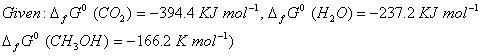Q. 17. At 300° C the thermal dissociation of HI is found to be 20%. What will be the equilibrium concentrations of H2 and l2 in the system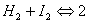at this temperature if the equilibrium concentration of HI in it be 0.96 mol L-1 ? (3)

Q. 18. Write three distinct differences between physical adsorption and chemisorption. (3)

Q. 19. Assign reasons for the following observations (3)

1. Mn2+ compounds are more stable than Fe2+ compounds towards oxidation to their +3 state.
2. Interstitial compounds are well known for transition elements.
3. An aqueous solution of potassium chromate is yellow but changes its colour on decreasing the pH of the solution.

Q. 20. Write the name (IUPAC norm) and draw the possible optical isomers of [CrCl2 (en) (NH3)2] + . (3)

Q. 21. Complete the following nuclear reaction symbolisations (3)

1.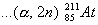2.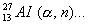3.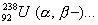(Note: you can put ‘X’ if the exact unknown symbol is not known)

Q. 22. Describe the following giving a chemical equation for each: (3)

1. Cannizzaro’s reaction
2. Transesterification
3. Hofmann bromamide reaction

Q. 23. Answer the following giving reaction conditions and a complete chemical equation in each case: (3)

1. How would you prepare ethyl amine from acetaldehyde?
2. How can you get benzonitrile from aniline?
3. How is ethyl isonitrile obtained from ethyl amine?

Q. 24. Describe the following giving a suitable example in each case: (3)

1. Antioxidants
2. Mordant dyes
3. Hybrid propellants

Q. 25.

1. Define the terms specific conductance and molar conductivity for solutions of electrolytes.
2. Write the cell formulation and calculate the standard cell potential of the galvanic cell in operation of which the following reaction takes place:
2 Cr (s) + 3 Cd2+ (aq) → 2 Cr 3+ (aq) + 3 Cd (s)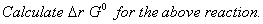(2, 3)Or

1. Explain with an example how weak and strong electrolytes can be distinguished.
2. In the button cell used in watches the following reaction occurs
Zn (s) + Ag2 O (s) + H2O (l) → Zn2+ (aq) + 2 Ag (s) + 2 OH-- (aq)
Determine E0 for the cell and ∆ r G0 for the reaction.
(Given: Zn2+ (aq) + 2e-- → Zn (s); E° = — 0.76 V
Ag2 O (s) +2 H2O (l)+ 2e-- →Ag (s) + 2 OH(aq),
E0 = + 0.34 V, F = 96500 C mol—1)

Q. 26.

1. How would you account for the following observations:
1. The tendency for catenation decreases as C> Si> Ge> Sn> Pb in Group 14 of the periodic table.
2. The pentavalent Bismuth is a strong oxidizing agent.
3. H3PO3 is a diprotic acid.
2. Draw the structural formulae of the following molecules:
1. Al2Cl 6 (s)
2. SF4 (g) (3, 2)

Or

1. Explain the following observations giving an appropriate reason in each case:
1. The +1 oxidation state of thallium is more stable than its +3 oxidation state.
2. SiCl62-- is not known to exist whereas SiF6 2- exists.
3. SF6 is inert towards hydrolysis.
2. Draw the structural formulae of the following molecules:
1. P4 O10 (s)
2. XeO3 (s)

Q. 27.

1. What are the light and the dark stages in photosynthesis in green plants? Give the basic equation of photosynthesis.
1. Which forces are responsible for the stability of ά-helix?
2. What is a denatured protein? (3, 2)

Or

1. What are reducing and non-reducing sugars? What is the structural feature characterising reducing sugars? What is invert sugar?
2. Define enzymes. What is the most important reason for their specificity in action?

 Chemistry 2006 Question Papers Class XII Delhi Outside Delhi Compartment Delhi Compartment Outside DelhiSet ISet ISet ISet I

 CBSE 2006 Question Papers Class XIIEnglishSociologyFunctional EnglishPsychologyMathematicsPhilosopyPhysicsComputer ScienceChemistryEntrepreneurshipBiologyInformatics PracticesGeographyMultimedia & Web TechnologyEconomicsBiotechnologyBusiness StudiesPhysical EducationAccountancyFine ArtsPolitical ScienceHistoryAgriculture